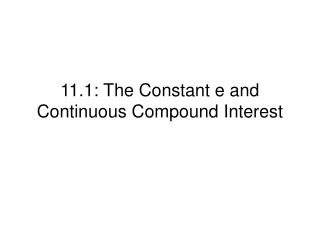DownloadDownload Presentation11.1: The Constant e and Continuous Compound Interest

# 11.1: The Constant e and Continuous Compound Interest

Download Presentation## 11.1: The Constant e and Continuous Compound Interest

- - - - - - - - - - - - - - - - - - - - - - - - - - - E N D - - - - - - - - - - - - - - - - - - - - - - - - - - -
##### Presentation Transcript

1. 11.1: The Constant e and Continuous Compound Interest

2. Review (Mat 115) • Just like π, e is an irrational number which can not be represented exactly by any finite decimal fraction. • However, it can be approximated by for a sufficiently large x e e e

3. The Constant e Reminder: Use your calculator, e = 2.718 281 828 459 … DEFINITION OF THE NUMBER e

4. Check the limit using table and graph

5. Review • Simple interest: A = P + Prt • Compound Interest: A = P(1 + r)t or with n = 1 (interest is compounded annually – once per year) • Other compounding periods: semiannually(2), quarterly(4), monthly(12), weekly(52), daily(365), hourly(8760)… • Continuous Compounding:(see page 589 for the proof) A = Pert A: future value P: principal r: interest rate t: number of years

6. Example 1: Generous Grandma Your Grandma puts \$1,000 in a bank for you, at 5% interest. Calculate the amount after 20 years. Simple interest: A = 1000 (1 + 0.0520) = \$2,000.00 Compounded annually: A = 1000 (1 + .05)20 =\$2,653.30 Compounded daily: Compounded continuously: A = 1000 e(.05)(20) = \$2,718.28

7. Example 2: IRA • After graduating from Barnett College, Sam Spartan landed a great job with Springettsbury Manufacturing, Inc. His first year he bought a \$3,000 Roth IRA and invested it in a stock sensitive mutual fund that grows at 12% a year, compounded continuously. He plans to retire in 35 years. • What will be its value at the end of the time period? • A = Pert = 3000 e(.12)(35) =\$200,058.99 • The second year he repeated the purchase of an identical Roth IRA. What will be its value in 34 years? • A = Pert = 3000 e(.12)(34) =\$177,436.41

8. Example 3 What amount (to the nearest cent) will an account have after 5 years if \$100 is invested at an annual nominal rate of 8% compounded annually? Semiannually? continuously? • Compounded annually • Compounded semiannually • Compounded continuously A = Pert = 100e(.08*5) = 149.18

9. If \$5000 is invested in a Union Savings Bank 4-year CD that earns 5.61% compounded continuously, graph the amount in the account relative to time for a period of 4 years. Example 4 • Use your graphing calculator: • Press y= • Type in 5000e^(x*0.0561) • Press ZOOM, scroll down, then press ZoomFit • You will see the graph • To find out the amount after 4 years • Press 2ND, TRACE, 1:VALUE • Then type in 4, ENTER

10. How long will it take an investment of \$10000 to grow to \$15000 if it is invested at 9% compounded continuously? Formula: A =P ert 15000 = 10000 e .09t 1.5 = e .09t Ln (1.5) = ln (e .09t) Ln (1.5) = .09 t So t = ln(1.5) / .09 t = 4.51 It will take about 4.51 years Example 5

11. How long will it take money to triple if it is invested at 5.5% compounded continuously? Formula: A =P ert 3P = P e .055t 3 = e .055t Ln 3 = ln (e .055t) Ln 3 = .055t So t = ln3 / .055 t = 19.97 It will take about 19.97 years Example 6

12. Review on how to solve exponential equations that involves e if needed (materials in MAT 115)